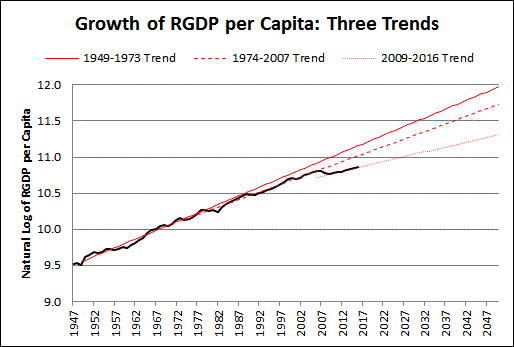## Thursday, July 6, 2017

###Real GDP per Capita growth: Three Trends

The previous post shows Real GDP with lines that represent growth trends for three different time periods. The topic of the post was the relation between economic growth and population growth.

But come to think of it, RGDP varies when population varies, and a change in population growth would change the trend of RGDP. So I want to take another look at the changes in Real GDP growth trends, this time excluding the changes in population. To do it, I'll look at Real GDP per Capita.

I'll make some other changes, too. Rather than using "billions of 2009 dollars" and showing the numbers on a "log scale", I'll get "log values" from FRED and plot them normally. That eliminates the Log Scale Axis Labels problem I had last time. And because the values are logged, the trends will be straight lines rather than exponential curves. So I can use Excel's "linear" trendline option and keep things nice and simple.

I'll use annual values instead of quarterly, to reduce the work involved. The purpose of the graph is to get a general idea about how the trends have changed, and the annual values are good enough for that. Annual frequency with "end of period" aggregation because, for GDP, it just seems right.

Here's my data source:Graph #1: Natural Log of Real GDP per Capita, 1947-2016
In order to define trend regions, I divided the dataset into three sections. The break points between sections are so arbitrary they made me hesitate -- hesitate enough to miss a day's posting. But then, missing a day's posting got me working again, "arbitrary" be damned.

I'm ending the first section at 1973 and starting the second at 1974. I'm ending the second section at 2007 and starting the third at 2009. In each case the section ends at a relative high value and the next section begins at a relative low. To be consistent, I started the first section at the relative low in 1949.

Because of these decisions, not all the data points are used to determine the trends. The data points not used are shown in black on the following graph; the points that are used create three separate red lines which represent the source data for the three trend calculations:Graph #2: Subsetting the Data for Determination of Trends
Once the data was split up into subsets, adding trend lines was easy.

For the next graph, the data not used is not shown. The data that is used is shown in black. And the trend lines are red. The year values are not shown, as adding those values messes up the trendline equations:Graph #3: Determining the Trends
This one's a little hard to read because the trend lines are too long. I'll fix that, below.

The equation for the first period (1949-1973) shows a slope of 0.024, indicating 2.4% annual growth. For the second period (1974-2007) the equation indicates 2.1% annual growth. And for the third period (2009-2016) the equation indicates a 1.34% annual growth rate.

For both the second and third periods the R-Squared value is 0.9889, pretty close to 1.0. For the first period, R-Squared is somewhat lower, at 0.9571. As I understand it, this means the first-period trend line is somewhat less accurate than the other two. The trend line doesn't match the data as well.

I want to suggest a reason for the mismatch: The first period was not part of the so-called "Great Moderation". The Great Moderation was a time of moderate variability in the data. (The "standard deviation" was less.) With less variability in the source data of the later periods, the trend line should be a better match. And we find that it is. So I think the lower R-Squared of the first period is a result of the fact that the data values varied more in that period, and not that there is something wrong with the trend.I took the three trendline equations from Graph #3 and changed them to display more decimal places, for greater accuracy when I use those equations to create my own lines. Making my own lines to show the trends gives me greater control over where the lines start and end. Also, I can show the years on the x-axis without messing up the trends.Graph #4: Three Trends of RGDP per Capita
This graph looks lot like the "three trends" graph for Real GDP in the previous post. But this graph shows that even when the changes in population are set aside, long-term decline of economic growth is evident.

// The Excel file